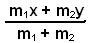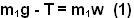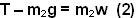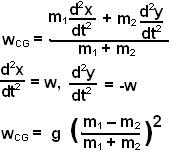Tuesday, May 6, 2008

Irodov Problem 1.143Suppose that at some instant masses m1 and m2 are x and y units below the pulley. The center of mass will be located,. Let the acceleration of the mass be w.

There are two forces acting on m1 , i) the tension pulling the mass up and ii) the force of gravity pulling it down and so we have,Similarly for mass m2 we can write,From (1) and (2) we have,

The acceleration of the CG will be,